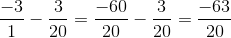## Example Questions

### Example Question #1 : How To Subtract Complex Fractions

Simplify,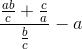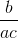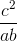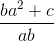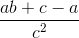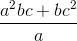Explanation:

Convert the numerators and denominators into single fractions, then simplify.

Start by finding the lowest common denominator in both the numerator and denominator of the complex fraction.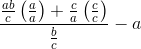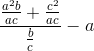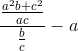Simplify. Divide complex fractions by multiplying the numerator by the reciprocal of the denominator.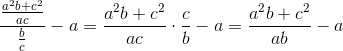Solve.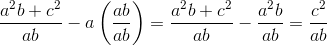### Example Question #2 : How To Subtract Complex Fractions

Subtract: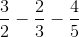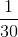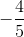Explanation:

The least common multiple can be found by multiplying the denominators: 2, 3, and 5. The common denominator of these numbers is 30. Multiply the numerator with what was multiplied to the denominator of each term, and then solve.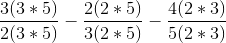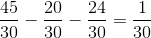### Example Question #3 : How To Subtract Complex Fractions

What is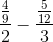?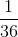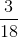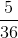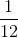Explanation:

First, simplify both sides.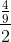becomes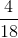and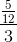becomes. The LCF betweenandis 36. Thus,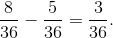This simplifies to.

### Example Question #4 : How To Subtract Complex Fractions

Simplify: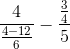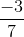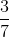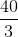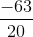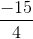Explanation:

Begin by simplifying the denominator of the first fraction: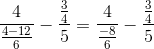Now, remember that division of fractions is done by multiplying the numerator by the reciprocal of the denominator. Thus: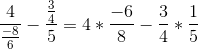Simplify a bit: